From walking to zipping, Part 2: Down and up

2008-08-17 19:19

The first post in this series showed what walks are and how to walk right through a value. This second post will add walking down into and up out of a value. Again, you can download this post as a program.

``````{-# OPTIONS -W -fglasgow-exts #-}
module WalkZip2 where
import WalkZip1
import Data.Generics (Data)
import Data.Char (isSpace)```
```

There are two ways to visualize a traversal.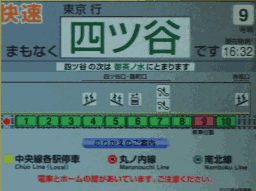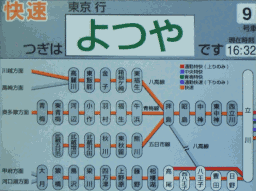The local way is to show the incoming and outgoing directions for a single step. So far we have just one incoming direction, `Before`, and just one outgoing direction, `After`.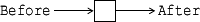The global way to visualize a traversal is to show how its steps connect parts of a whole. So far all we can do is to forge right.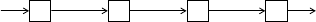To traverse a value with hierarchical structure, we want to descend from a parent part to visit its children, and to ascend from a child part to admire its parent. Therefore, locally speaking, we add one incoming direction and one outgoing direction.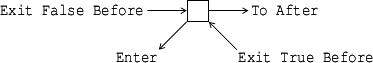This way we connect a parent with its children as shown in the following global picture. In the top row are the parent and its siblings. In the bottom row are the children. The ellipses indicate the children’s cousins and own children.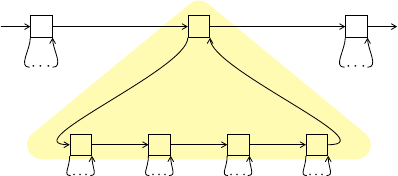The first time we visit a parent, we come in from its left. We can choose to skip the children by moving right or to visit the children by moving down. If we choose the latter, then after visiting all the children, we return to the parent from below, and can choose to move right or descend again. This loop is shaded in the global picture above.

As the local picture above shows, we rename the old directions as we add the new directions. Each incoming direction `from`—there is only one so far, namely `Before`—becomes two incoming directions, ```Exit False from``` and `Exit True from`. The latter direction means that we just emerged from visiting the last child in a horizontal sequence.

``````data Exit from = Exit Bool from
```

Each outgoing direction `to`—there is only one so far, namely `After`—becomes an outgoing direction `To to` alongside the new outgoing direction `Enter`, which means to descend to the first child of the current part.

``````data Enter to = Enter | To to
```

Every time a visitor arrives at a part, the walk orients the visitor by passing an incoming direction. Every time a visitor departs from a part, the visitor directs the walk by returning an outgoing direction. In particular, if the visitor says to go down (`Enter`) but there is no child to visit, the walk stays at the same location and tells the visitor that there is no child to visit by passing the incoming direction ```Exit True Before```. Indeed, as the last picture indicates, the way to go up is to keep going right until you arrive at a part from below.

That’s the plan. Let’s code it up. First we lift the incoming direction `before` from any type `from` to the type `Exit from`.

``````instance (From from) => From (Exit from) where
before = Exit False before```
```

We also lift the outgoing direction `after` from any type `to` to the type `Enter to`.

``````instance (To to) => To (Enter to) where
after = To after```
```

To conduct recursive tourism, we define `next` to descend once into everything. That is, descend when we arrive at a part from the left, but continue to the right when we arrive at a part from below.

``````instance (Next Before to)
=> Next (Exit Before) (Enter to) where
next (Exit False Before) = Enter
next (Exit True  Before) = To after```
```

We now build a walk combinator `around`, of the following type.

``````around :: Walk from to part whole
-> Walk (Exit from) (Enter to) part part
-> Walk (Exit from) (Enter to) part whole```
```

This combinator takes two walks `walkOuter` and `walkInner` as argument and produces a new walk. In the last picture, the top row corresponds to `walkOuter` and the bottom row corresponds to `walkInner`. Whereas `walkOuter` steps through parts of a whole, `walkInner` steps through (sub)parts of a part. The new walk is like `walkOuter`, except at any step the visitor can `Enter` an inner walk to inspect the current part.

The code for `around` is straightforward. The boring part is how it uses the helper functions `scavenge` (from last time) and `pollute` (below) to do the `dirty` work of preserving sharing. The interesting part is how it builds a visitor `visit'` for the outer walk by wrapping around an inner walk that uses the original visitor `visit`.

``````around walkOuter walkInner visit
= walkOuter (visit' False False)
where visit' dirty around from part = do
(part1_, to) <- visit (Exit around from) part
let (dirty1, part1) = pollute dirty part part1_
case to of
Enter -> do
part2_ <- walkInner visit part1
let (dirty2, part2) = pollute dirty1 part1 part2_
visit' dirty2 True from part2
To to -> return (scavenge dirty1 part1, to)

pollute :: Bool -> a -> Maybe a -> (Bool, a)
pollute dirty a Nothing = (dirty, a)
pollute _ _ (Just a) = (True, a)```
```

To try this out, let me first define a New York walk. A New York walk makes no `stop`s, not even one.

``````newYork :: Walk from to part whole
newYork _ _ = return Nothing```
```

The New York walk is so brisk, no tourist gets to see anything or touch any button.

``````*WalkZip2> (newYork :: Walk Before After Term Term) tourist term
Nothing
*WalkZip2> (newYork :: Walk Before After Term Term) tourist' term
Nothing```
```

If we use the New York walk as the inner walk in `around`, then no part has any subpart, so a visitor who tries to enter a part is whisked right out.

``````*WalkZip2> (stop Before `around` newYork) tourist term
Exit False Before: A (L "x" (A (V "x") (V "x"))) (L "x" (A (V "x") (V "x")))
Exit True Before: A (L "x" (A (V "x") (V "x"))) (L "x" (A (V "x") (V "x")))
Nothing```
```

But if we put this walk in an inner walk, then a part can have subparts, though a subpart cannot have subsubparts, so a visitor who tries to enter a subpart is whisked out.

``````*WalkZip2> (stop Before `around` gwalk (stop Before `around` newYork)) tourist term
Exit False Before: A (L "x" (A (V "x") (V "x"))) (L "x" (A (V "x") (V "x")))
Exit False Before: L "x" (A (V "x") (V "x"))
Exit True Before: L "x" (A (V "x") (V "x"))
Exit False Before: L "x" (A (V "x") (V "x"))
Exit True Before: L "x" (A (V "x") (V "x"))
Exit True Before: A (L "x" (A (V "x") (V "x"))) (L "x" (A (V "x") (V "x")))
Nothing```
```

To go one level further into the term, we can put this last walk in an inner walk, so that only a subsubpart cannot have subsubsubparts.

``````*WalkZip2> (stop Before `around` gwalk (stop Before `around` gwalk (stop Before `around` newYork))) tourist term
Exit False Before: A (L "x" (A (V "x") (V "x"))) (L "x" (A (V "x") (V "x")))
Exit False Before: L "x" (A (V "x") (V "x"))
Exit False Before: A (V "x") (V "x")
Exit True Before: A (V "x") (V "x")
Exit True Before: L "x" (A (V "x") (V "x"))
Exit False Before: L "x" (A (V "x") (V "x"))
Exit False Before: A (V "x") (V "x")
Exit True Before: A (V "x") (V "x")
Exit True Before: L "x" (A (V "x") (V "x"))
Exit True Before: A (L "x" (A (V "x") (V "x"))) (L "x" (A (V "x") (V "x")))
Nothing```
```

To walk through all nodes of a tree, we take the fixpoint of this process.

``````throughout :: (Data a) => Walk from to a a
-> Walk (Exit from) (Enter to) a a
throughout level = level `around` gwalk (throughout level)```
```

Whee!

``````*WalkZip2> throughout (stop Before) tourist term
Exit False Before: A (L "x" (A (V "x") (V "x"))) (L "x" (A (V "x") (V "x")))
Exit False Before: L "x" (A (V "x") (V "x"))
Exit False Before: A (V "x") (V "x")
Exit False Before: V "x"
Exit True Before: V "x"
Exit False Before: V "x"
Exit True Before: V "x"
Exit True Before: A (V "x") (V "x")
Exit True Before: L "x" (A (V "x") (V "x"))
Exit False Before: L "x" (A (V "x") (V "x"))
Exit False Before: A (V "x") (V "x")
Exit False Before: V "x"
Exit True Before: V "x"
Exit False Before: V "x"
Exit True Before: V "x"
Exit True Before: A (V "x") (V "x")
Exit True Before: L "x" (A (V "x") (V "x"))
Exit True Before: A (L "x" (A (V "x") (V "x"))) (L "x" (A (V "x") (V "x")))
Nothing```
```

You might be tired of all this recursive tourism where the visitor just follows a predetermined route and makes no choice. I certainly much prefer interactive decision-making over horseback flower-watching! To finish up this post, let’s control a walk from the keyboard. The following visitor is convenient for that: it prompts us at each step for what to return. A blank line means to take the `next` step without making any changes.

``````keyboard :: (Next from to, Show a, Read a)
=> from -> a -> IO (Maybe a, to)
keyboard from x = do
putStr (show from ++ ": " ++ show x ++ "\n? ")
line <- getLine
return (if all isSpace line
then (Nothing, next from)
```

Let’s stroll through the term (λx.xx)(λx.xx) and change its second part to (λx.x), then double back to review the new term (λx.xx)(λx.x).

``````*WalkZip2> throughout (stop Before) keyboard term
Exit False Before: A (L "x" (A (V "x") (V "x"))) (L "x" (A (V "x") (V "x")))
?
Exit False Before: L "x" (A (V "x") (V "x"))
? (Nothing, To After)
Exit False Before: L "x" (A (V "x") (V "x"))
?
Exit False Before: A (V "x") (V "x")
? (Just (V "x"), Enter)
Exit True Before: V "x"
?
Exit True Before: L "x" (V "x")
?
Exit True Before: A (L "x" (A (V "x") (V "x"))) (L "x" (V "x"))
? (Nothing, Enter)
Exit False Before: L "x" (A (V "x") (V "x"))
?
Exit False Before: A (V "x") (V "x")
? (Nothing, To After)
Exit True Before: L "x" (A (V "x") (V "x"))
? (Nothing, To After)
Exit False Before: L "x" (V "x")
? (Nothing, To After)
Exit True Before: A (L "x" (A (V "x") (V "x"))) (L "x" (V "x"))
? (Nothing, To After)
Just (A (L "x" (A (V "x") (V "x"))) (L "x" (V "x")))```
```

In the next post, we will take a walk outside the `IO` monad, to make a zipper.